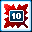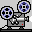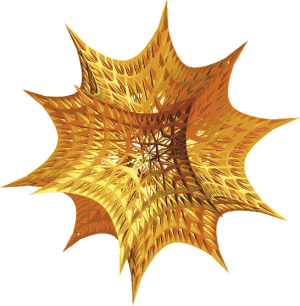# Analytic, Geometric, and Numeric Analysis of the Shrinking Circle and Sphere Problems

### Douglas B. Meademeade@math.sc.eduDepartment of MathematicsUniversity of South Carolina Columbia, SC 29208 USA

Abstract

The Shrinking Circle Problem is an example of a simple-to-state geometry problems can be visually appealing yet quite challenging to solve. A combination of geometry and analysis is used to completely solve the general problem in the plane, and its extension to three dimensions: the Shrinking Sphere Problem. We show why traditional numerical attempts to answer even the simplest problem is futile. The general results were first formulated based on visual evidence produced by dynamic geometry software. Only then was it possible to utilize symbolic computation tools to put together the complete proofs. All supplemental materials that accompany this paper can be found at the URL

## Supplemental Resources by Section

Video Sketch
Geom
Expr
Maple
10
HTML
§1 - IntroductionHTML Geometric Solution of the Original Shrinking Circle Problem
§2 - The Original Shrinking Circle ProblemHTML Analytic Solution of the Original Shrinking Circle ProblemHTML Numeric Solution of the Original Shrinking Circle Problem (Table 1)
§3 - The Generalized Shrinking Circle ProblemHTML Lemma 1
Derivations and Animations for Other Fixed CurvesHTML EllipseHTML LineHTML ParabolaTheorem 2
§4 - The Simplest Shrinking Sphere ProblemsHTML Lemma 4 (including Figure 4)
§5 - The Generalized Shrinking Sphere Problems
Derivations and Animations for Other Fixed SurfacesHTML EllipsoidHTML ConeHTML Paraboloid
§6 - Discussion of Numerical Limits and VisualizationNumerical Difficulties for the Original Shrinking Circle ProblemHTML Visualizing the Generalized Shrinking Sphere with Discrete Samples (Figure 5)Visualizing the Generalized Shrinking Sphere with Geometric Objects (Figure 6)
§7 - Conclusion

## List of SoftwareGeometry Expressions http://www.geometryexpressions.com/Geometer's Sketchpad http://www.dynamicgeometry.com/Maple http://www.maplesoft.com/Mathematica http://www.wolfram.com/